Courses
Courses for Kids
Free study material
Free LIVE classes
More

# Dot PlotLIVE
Join Vedantu’s FREE Mastercalss

## Dot Plot Meaning

A dot plot, also known as a dot diagram, is a statistical chart consisting of data points on a relatively simple scale. Dot plots are considered as one of the easiest statistical plots, used for small data sets. Dot plots are used for highlighting clusters, gaps, skews in distribution, and outliers. When working with datasets larger than 20 or 30, it is better to use another statistical chart such as a histogram, as the dot plot will be less manageable.

## Constructing a Dot Diagram

While constructing a dot diagram, data points are stacked in a column over a category. The height of the column denotes the frequency of observation in a given category. The categories of dot diagram given below are the numbers from 0 to 9, where 0, 1, and 5 occur three times, 2 occurs 7 times, 3 and 4 occurs six times, 6 occurs 4 times, 7 and 9 occurs 9 times, and 8 occurs two times.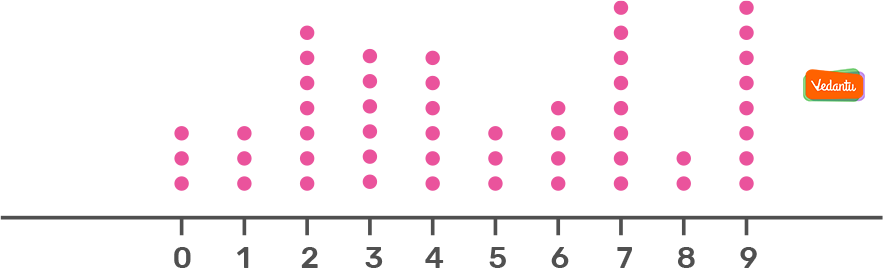The dot diagram given above represents the total number of data points and how the data points are distributed among certain categories.

## Analyzing Dot Plot

A dot plot or dot diagram consists of a horizontal scale (a number line) on which dots are arranged to represent the numerical values of the data set. If the values in a data set repeat the dots are accumulated at that location. One dot is plotted for each repetition. We can say that dot diagrams represent the distribution of data.

Analyzing the dot plot of a given data set can be done easily in comparison to a long list of numbers. By looking at the dot plot diagram given below, you can see that dots are evenly spread with a peak at around 8 letters. The centre of the graph is between 6 and 7 letters. The dots are spread from 3 to 9 letters.

With a dot plot, you can easily count the number of times each category, or number is repeated. A dot plot is an optimum way of organizing numbers and values.In a dot plot, you can easily count the number of times each category, or number is repeated. A dot plot is an optimum way of organizing numbers and values.

## Dot Plot Interpretation

As you know, a dot plot is made up of dots plotted on a graph. Considering this, let us learn dot plot interpretation.

• Each dot plotted in a dot graph denotes a specific number of observations from a given set of data (unless specified, assume that each dot represents one observation. If a dot represents more than one observation, that should be written on the plot.

• The dots are arranged in a column over category, so that height of the column represents the absolute or relative frequency of observation in the category.

• The pattern of data formed in a dot plot can be clearly stated in terms of symmetry and skewness only if the categories are quantitative, but if the categories are qualitative, a dot plot is not suitable to describe those terms.

In comparison to other types of graphs, dot plots are often used to plot frequency counts within a small number of categories, generally a small set of data.

## Types of Dot Plot

There are two types of dot plot namely:

1. Wilkinson Dot Plot

2. Cleveland Dot Plot

### Wilkinson Dot Plot

The Wilkinson Dot Plot represents the distribution of continuous data points, like a histogram. For example, the tempurature in a room throughout the day.

A histogram is shown in Wilkinson Dot Plot but represents the individual data points like P, Q, R, S shown below rather than bins. These points can consider any values like Mathematics marks of four friends, or the number of minutes taken by four kids to finish their lunch.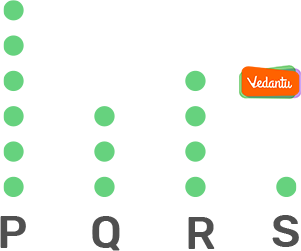### Cleveland Dot Plot

Cleveland dot plot is a good alternative to a simple bar chart, specifically if you have more than a few items.

It does not take much for a graph to look cluttered. In the same amount of space, many more values can be plotted in a dot plot and it is easier to read as well.

Although this type of dot plot is similar to a bar chart but uses location rather than the duration of the data encoding values.

Just like the height of the bar chart represents the number of items, the position of dots in the graph represents the number of items for that category or bin.

For example, the Cleveland dot plot given below represents the trend in literacy rate in Post independent India.

For example,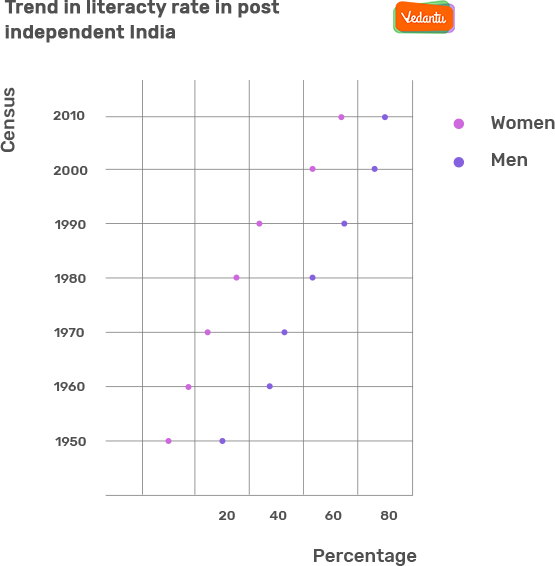### Dot Plot Examples

1. The dot plot given below represents the number and types of pizzas ordered for the party.  By analyzing the dot plot, determine the number of pizzas ordered for the party, How many of these pizzas were pepperoni or cheese?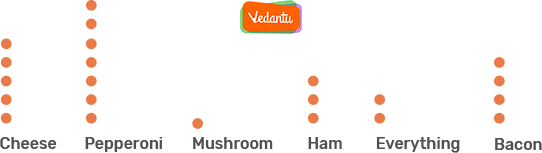Solution:

To determine the number of pizzas ordered for the party, add all of the data points together on a dot plot. In total, 22 pizzas were ordered for the party.

To find the numbers of pizzas ordered for a certain category, sum up all the points in those categories. There are 7 pepperoni pizzas and 5 cheese pizzas. In total 12 pizzas are of cheese or pepperoni.

2. The dot plot given below represents the height of each kid at Mr. George's daycare. Each dot represents the height of different kids. Determine the height of the shortest kid.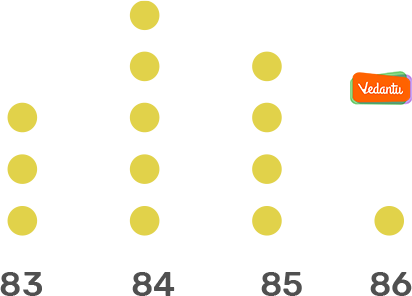Solution:

The height range of kids on the axis is from 83 - 86.

The 3 dots are plotted on bin - 83 which implies that there are 3 kids whose height is 83.

Therefore, the height of the shortest kid is 83.

Last updated date: 21st Sep 2023
Total views: 282.6k
Views today: 6.82k

## FAQs on Dot Plot

Q1. Where the Dot Plot is Used for?

Ans. A dot plot is a graphical representation of data using dots and a simple scale to make the comparison of frequencies within groups or categories.

Dot plots are used for quantitative, continuous, and univariate data.  It is used to convey important information to the viewers using the dots or it can be used in school and college to display any data.

Dot plots are easy to construct so it is used in most of the places to display the information. Dot plots are useful for highlighting gaps or clusters.

Q2. What are the Pros and Cons of the Dot Plot?

Ans. Dot plots can be speedily prepared for small sets of data. They don't require frequency and offer a visual means of comparison. However, in a dot plot, you need to count the total number of data elements in each stack of dots, and it can be difficult to construct and interpret data sets for many points.

Q3. Why are Dot Plots More Preferred Than Histograms?

Ans. A histogram is a chart that groups the data into classes and represents the information of those frequencies while a dot plot represents each observation on a number line.

In dot, the identity of individual observation is not lost while in histograms the identity of each observation is lost as data are grouped into classes and then plotted.

The dot plot is more appropriate than the histogram for small data set.